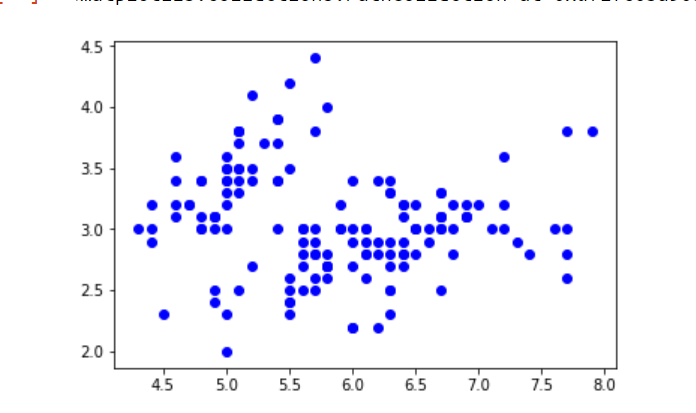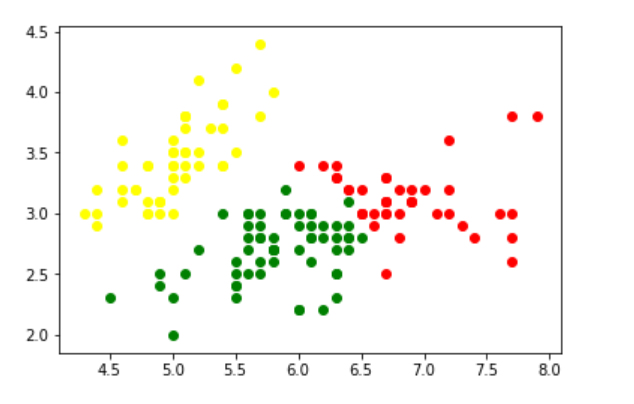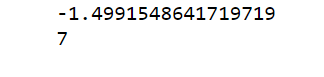# Gaussian Mixture Model

Suppose there are set of data points that needs to be grouped into several parts or clusters based on their similarity. In machine learning, this is known as Clustering.

There are several methods available for clustering like:

### Normal or Gaussian Distribution

In real life, many datasets can be modeled by Gaussian Distribution (Univariate or Multivariate). So it is quite natural and intuitive to assume that the clusters come from different Gaussian Distributions. Or in other words, it is tried to model the dataset as a mixture of several Gaussian Distributions. This is the core idea of this model.

In one dimension the probability density function of a Gaussian Distribution is given bywhereandare respectively mean and variance of the distribution.

For Multivariate ( let us say d-variate) Gaussian Distribution, the probability density function is given byHereis a d dimensional vector denoting the mean of the distribution andis the d X d covariance matrix.

### Gaussian Mixture Model

Suppose there are K clusters (For the sake of simplicity here it is assumed that the number of clusters is known and it is K). Soandis also estimated for each k. Had it been only one distribution, they would have been estimated by maximum-likelihood method. But since there are K such clusters and the probability density is defined as a linear function of densities of all these K distributions, i.e.whereis the mixing coefficient for k-th distribution.

For estimating the parameters by maximum log-likelihood method, compute p(X|,,).Now define a random variablesuch that=p(k|X).

From Bayes’theorem,Now for the log likelihood function to be maximum, its derivative ofwith respect to,andshould be zero. So equaling the derivative ofwith respect toto zero and rearranging the terms,Similarly taking derivative with respect toandrespectively, one can obtain the following expressions.AndNote:denotes the total number of sample points in the k-th cluster. Here it is assumed that there are total N number of samples and each sample containing d features are denoted by.

So it can be clearly seen that the parameters cannot be estimated in closed form. This is where Expectation-Maximization algorithm is beneficial.

### Expectation-Maximization (EM) Algorithm

The Expectation-Maximization (EM) algorithm is an iterative way to find maximum-likelihood estimates for model parameters when the data is incomplete or has some missing data points or has some hidden variables. EM chooses some random values for the missing data points and estimates a new set of data. These new values are then recursively used to estimate a better first data, by filling up missing points, until the values get fixed.
These are the two basic steps of the EM algorithm, namely E Step or Expectation Step or Estimation Step and M Step or Maximization Step.

• Estimation step:
• initialize,andby some random values, or by K means clustering results or by hierarchical clustering results.
• Then for those given parameter values, estimate the value of the latent variables (i.e)
• Maximization Step:
• Update the value of the parameters( i.e.,and) calculated using ML method.

Algorithm:


Initialize the mean,
the covariance matrixand
the mixing coefficientsby some random values. (or other values)
Compute thevalues for all k.
Again Estimate all the parameters
using the currentvalues.
Compute log-likelihood function.
Put some convergence criterion
If the log-likelihood value converges to some value
( or if all the parameters converge to some values )
then stop,


Example:
In this example, IRIS Dataset is taken. In Python there is a GaussianMixture class to implement GMM.

Note: This code might not run in an online compiler. Please use an offline ide.

1. Load the iris dataset from datasets package. To keep things simple, take only first two columns (i.e sepal length and sepal width respectively).
2. Now plot the dataset.

 import numpy as np  import pandas as pd  import matplotlib.pyplot as plt  from pandas import DataFrame  from sklearn import datasets  from sklearn.mixture import GaussianMixture     # load the iris dataset  iris = datasets.load_iris()     # select first two columns   X = iris.data[:, :2]     # turn it into a dataframe  d = pd.DataFrame(X)     # plot the data  plt.scatter(d, d)3. Now fit the data as a mixture of 3 Gaussians.
4. Then do the clustering, i.e assign a label to each observation. Also find the number of iterations needed for the log-likelihood function to converge and the converged log-likelihood value.

 gmm = GaussianMixture(n_components = 3)     # Fit the GMM model for the dataset   # which expresses the dataset as a   # mixture of 3 Gaussian Distribution  gmm.fit(d)     # Assign a label to each sample  labels = gmm.predict(d)  d['labels']= labels  d0 = d[d['labels']== 0]  d1 = d[d['labels']== 1]  d2 = d[d['labels']== 2]     # plot three clusters in same plot  plt.scatter(d0, d0, c ='r')  plt.scatter(d1, d1, c ='yellow')  plt.scatter(d2, d2, c ='g')5. Print the converged log-likelihood value and no. of iterations needed for the model to converge

 # print the converged log-likelihood value  print(gmm.lower_bound_)     # print the number of iterations needed  # for the log-likelihood value to converge  print(gmm.n_iter_)</div>6. Hence, it needed 7 iterations for the log-likelihood to converge. If more iterations are performed, no appreciable change in the log-likelihood value, can be observed.

Attention reader! Don’t stop learning now. Get hold of all the important CS Theory concepts for SDE interviews with the CS Theory Course at a student-friendly price and become industry ready.

My Personal Notes arrow_drop_upCheck out this Author's contributed articles.

If you like GeeksforGeeks and would like to contribute, you can also write an article using contribute.geeksforgeeks.org or mail your article to contribute@geeksforgeeks.org. See your article appearing on the GeeksforGeeks main page and help other Geeks.

Please Improve this article if you find anything incorrect by clicking on the "Improve Article" button below.